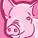# QlikView App Dev

Discussion Board for collaboration related to QlikView App Development.

Announcements
Skip the ticket, Chat with Qlik Support instead for instant assistance.
cancel
Showing results for
Did you mean:Anonymous
Not applicable

## SUM Multiple Fields

Dear experts

I am trying to derive a percentage using the Expression below, it will display in a text object (its a visual thing).

=num(sum([field 1])+sum([field 2])+sum([field 3])/sum([field 4]),'#0%'

I get the statement 'Expression OK' but the figure I get back is neither correct in value or formatted as a %.

I am new to this, so not yet familiar with the 'language' and common methodologies - using QV 11.2

Grateful for any guidance.

Jason.

1 Solution

Accepted SolutionsMVP

You can also try this:

=Num((Sum([field 1])+Sum([field 2])+Sum([field 3]))/Sum([field 4]),'#0%')

8 RepliesMVP

Maybe

=num( Rangesum(sum([field 1]),sum([field 2]),sum([field 3]) ) /sum([field 4]),'#0%')Anonymous
Not applicable
Author

Thanks Stefan - but this seems return the value of Field 1 + Field 2 only.MVP

You can also try this:

=Num((Sum([field 1])+Sum([field 2])+Sum([field 3]))/Sum([field 4]),'#0%')Anonymous
Not applicable
Author

Perfect - Thank you Sunny - I was warned it would all be in the brackets! 🙂

JasonMVP

Yes, or maybe even

=num(Sum( Rangesum( [field 1],[field 2],[field 3]) ) / sum([field 4]),'#0%')

Bottom line: Take care of multiplication / division operator precedes addition operator rule, use brackets or functions to get the sum of fields right before dividing it by the denominator.Anonymous
Not applicable
Author

Thank you for the additional method and sound advice Stefan, very much appreciated.MVP

Without knowing your data model (e.g. your fields are located in different tables) and data, it's hard to tell why this is happening.

But glad you got it working.Contributor

I found the following to work in QlikSense for me, using the "rangesum" replacing all "sum" and using "," instead of "+"

num(rangesum([field 1])/Rangesum(rangesum([field 2]),rangesum([field 3]),rangesum([field 4])) ,'#0%')Community Browser